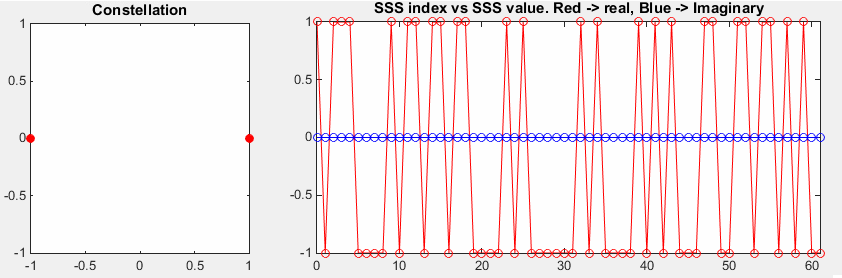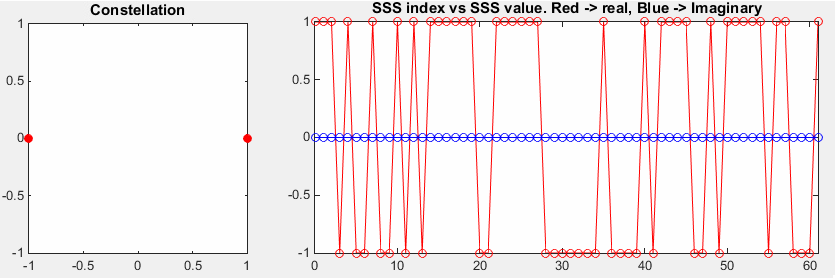MatLab Toolbox - LTE                                      Home : www.sharetechnote.com

Secondary Synchronization Signal (SSS)

If you don't know what SSS (Secondary Synchronization Signal), refer to Physical Layer Signal : SSS (Secondary Synchronization Signal) page first.

% Since PSS is determined by each eNodeB, you have to define properites of a eNodeB.

% NDLRB indicate System Bandwith in the unit of RBs.

% NDLRB 6 = 1.4 Mhz, NDLRB 15 = 3.0 Mhz, NDLRB 25 = 5.0 Mhz,

% NDLRB 50 = 10 Mhz, NDLRB 75 = 15 Mhz, NDLRB 100 = 20 Mhz

% CellRefP indicate number of downlink Antenna. CellRefP = 1 means 1 transmission antenna (SISO)

% NCellID indicate PCI (Physical Channel Identity) of the Cell

% NSubframe indicate the subframe number. Since PSS resides only in a specific subframe,

% you need to specify the proper subframe number here.

enb.CyclicPrefix = 'Normal';

enb.NDLRB = 6;

enb.CellRefP = 1;

enb.DuplexMode = 'FDD';

enb.NCellID = 0;

enb.NSubframe = 0;

% if you pass the eNodeB information (enb) into lteSSS(), it will generate OFDM symbols for the specified PSS.

% sss_arrayIndex is just a sequence of integer which will used to plot PSS symbols.

sss = lteSSS(enb);

sss_arrayIndex = 0:length(sss)-1;

% Following is to represent SSS symbols.

subplot(1,3,1);

plot(real(sss),imag(sss),'ro','MarkerFaceColor',[1 0 0]);

title('Constellation');

subplot(1,3,[2 3]);

plot(sss_arrayIndex,real(sss),'ro-',pss_arrayIndex,imag(sss),'bo-');

xlim([0 max(sss_arrayIndex)]);

title('SSS index vs SSS value. Red -> real, Blue -> Imaginary');

If you see the left plot (constellation), you would notice SSS is not based on  ZadOff Chu sequence. It would be a little difficult to understand the nature of these numbers just by looking at it. It is made by a kind of scrambling squence (based on m-sequence). As you see in the right side graph, SSS is made up of 62 symbols.

 enb.CellRefP = 1; enb.NCellID = 0;The only difference between this example and previous example is NCellID. If you compare this with previous example, you would notice that the sequence of symbols are different. It means SSS sequence varies with NCellID (PCI : Physical Cell ID)

 enb.CellRefP = 1; enb.NCellID = 1;The only difference between this example and previous example is NCellID. If you compare this with previous example, you would notice that the sequence of symbols are different. It means SSS sequence varies with NCellID (PCI : Physical Cell ID)

 enb.CellRefP = 1; enb.NCellID = 3;The only difference between this example and previous example is NCellID. If you compare this with previous example, you would notice that the sequence of symbols are different. It means SSS sequence varies with NCellID (PCI : Physical Cell ID)

 enb.CellRefP = 1; enb.NCellID = 168;Did you see any pattern from the various sequence of SSS shown above ? do you understand exactly how PCI influence the specific sequence generation of SSC ?

I know, it would be almost impossible to figure out the answers to these questions. Now it is time for you to get back to Physical Layer Signal : SSS (Secondary Synchronization Signal) again and look into details of the extremely confusing mathmatical formula. At least, I tried to link a those mathematical steps in such a way that you can follow up a little more easily than 3GPP spec itself (hopefully :))

Disclaimer ! :

This page is only to show you the overall logics and visualization for various LTE physical layer channels. I haven't investigated much about verifying about the accuracy.

If you think the code is not so efficient, it is 100% my fault. I haven't made any effort for effiecient code. I just tried to create code as simple as possible for the readers. As you know, easy-to-read code is not always efficient for a specific chipset.

If you find any mistake in terms of accuracy, it is also very highly likely be my fault. Not the problem of Matlab tool box itself.

Any comment and corrections if you find any mistake will be welcome and appreciated.